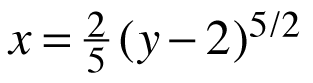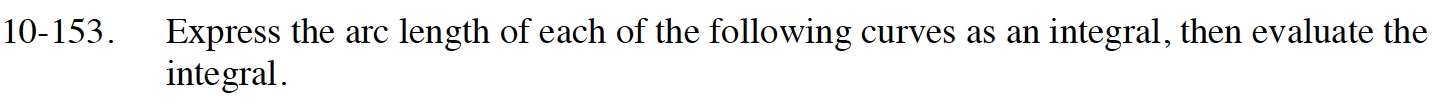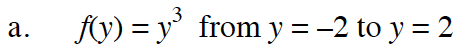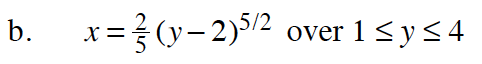### Home > CALC > Chapter 10 > Lesson 10.4.2 > Problem10-153

10-153.
1. Express the arc length of each of the following curves as an integral, then evaluate the integral. Homework Help ✎

1. f(y) = y3 from y = –2 to y = 2

2.between 1 ≤ y ≤ 4Don't let the notation confuse you. This is the same problem as calculating the arclength for f(x) = x3 from x = –2 to x = 2.$\text{arc length}=\int_1^4\sqrt{1+(x^\prime)^2}dy$